# Plot single trial activity, grouped by ROI and sorted by RT¶

This will produce what is sometimes called an event related potential / field (ERP/ERF) image.

The EEGLAB example file, which contains an experiment with button press responses to simple visual stimuli, is read in and response times are calculated. Regions of Interest are determined by the channel types (in 10/20 channel notation, even channels are right, odd are left, and ‘z’ are central). The median and the Global Field Power within each channel group is calculated, and the trials are plotted, sorting by response time.

```# Authors: Jona Sassenhagen <jona.sassenhagen@gmail.com>
#

import mne
from mne.event import define_target_events
from mne.channels import make_1020_channel_selections

print(__doc__)
```

Load EEGLAB example data (a small EEG dataset)

```data_path = mne.datasets.testing.data_path()
fname = data_path + "/EEGLAB/test_raw.set"

event_id = {"rt": 1, "square": 2}  # must be specified for str events

mapping = {
'EEG 000': 'Fpz', 'EEG 001': 'EOG1', 'EEG 002': 'F3', 'EEG 003': 'Fz',
'EEG 004': 'F4', 'EEG 005': 'EOG2', 'EEG 006': 'FC5', 'EEG 007': 'FC1',
'EEG 008': 'FC2', 'EEG 009': 'FC6', 'EEG 010': 'T7', 'EEG 011': 'C3',
'EEG 012': 'C4', 'EEG 013': 'Cz', 'EEG 014': 'T8', 'EEG 015': 'CP5',
'EEG 016': 'CP1', 'EEG 017': 'CP2', 'EEG 018': 'CP6', 'EEG 019': 'P7',
'EEG 020': 'P3', 'EEG 021': 'Pz', 'EEG 022': 'P4', 'EEG 023': 'P8',
'EEG 024': 'PO7', 'EEG 025': 'PO3', 'EEG 026': 'POz', 'EEG 027': 'PO4',
'EEG 028': 'PO8', 'EEG 029': 'O1', 'EEG 030': 'Oz', 'EEG 031': 'O2'
}
raw.rename_channels(mapping)
raw.set_channel_types({"EOG1": 'eog', "EOG2": 'eog'})
raw.set_montage('standard_1020')

events = mne.events_from_annotations(raw, event_id)
```

Out:

```Reading /home/circleci/mne_data/MNE-testing-data/EEGLAB/test_raw.fdt
Used Annotations descriptions: ['rt', 'square']
```

Create Epochs

```# define target events:
# 1. find response times: distance between "square" and "rt" events
# 2. extract A. "square" events B. followed by a button press within 700 msec
tmax = 0.7
sfreq = raw.info["sfreq"]
reference_id, target_id = 2, 1
new_events, rts = define_target_events(events, reference_id, target_id, sfreq,
tmin=0., tmax=tmax, new_id=2)

epochs = mne.Epochs(raw, events=new_events, tmax=tmax + 0.1,
event_id={"square": 2})
```

Out:

```Not setting metadata
73 matching events found
Setting baseline interval to [-0.203125, 0.0] sec
Applying baseline correction (mode: mean)
0 projection items activated
```

Plot using global field power

```# Parameters for plotting
order = rts.argsort()  # sorting from fast to slow trials

selections = make_1020_channel_selections(epochs.info, midline="12z")

# The actual plots (GFP)
epochs.plot_image(group_by=selections, order=order, sigma=1.5,
overlay_times=rts / 1000., combine='gfp',
ts_args=dict(vlines=[0, rts.mean() / 1000.]))
```
•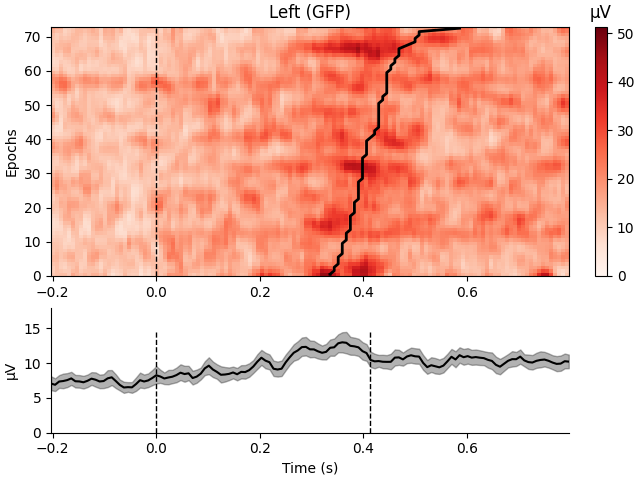•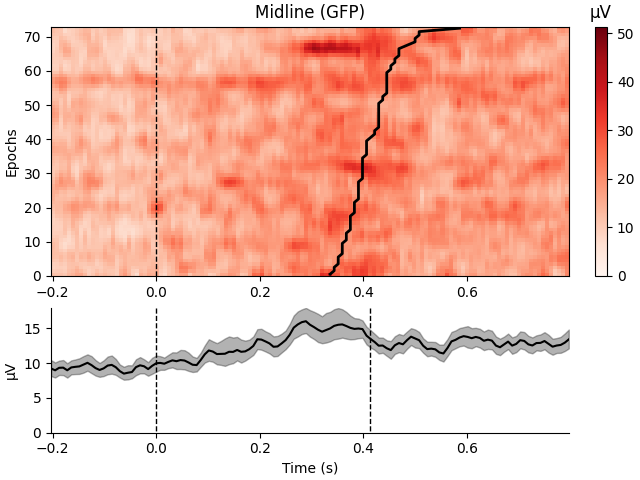•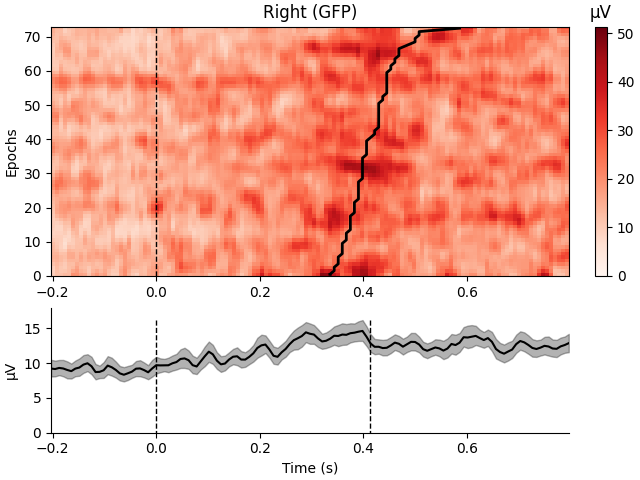Out:

```Loading data for 73 events and 129 original time points ...
73 matching events found
No baseline correction applied
0 projection items activated
73 matching events found
No baseline correction applied
0 projection items activated
73 matching events found
No baseline correction applied
0 projection items activated
combining channels using "gfp"
combining channels using "gfp"
combining channels using "gfp"
```

Plot using median

```epochs.plot_image(group_by=selections, order=order, sigma=1.5,
overlay_times=rts / 1000., combine='median',
ts_args=dict(vlines=[0, rts.mean() / 1000.]))
```
•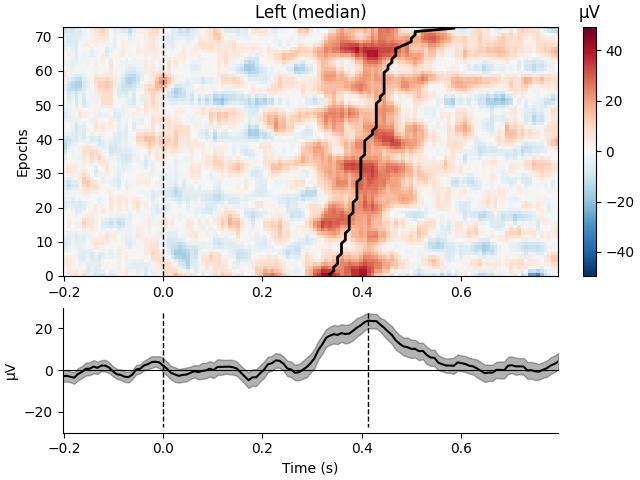•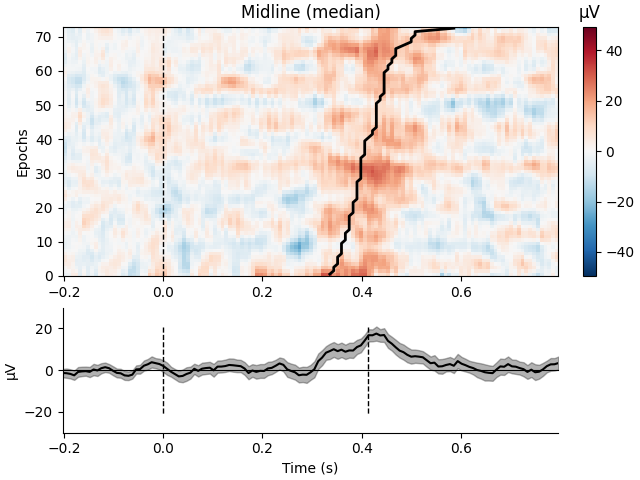•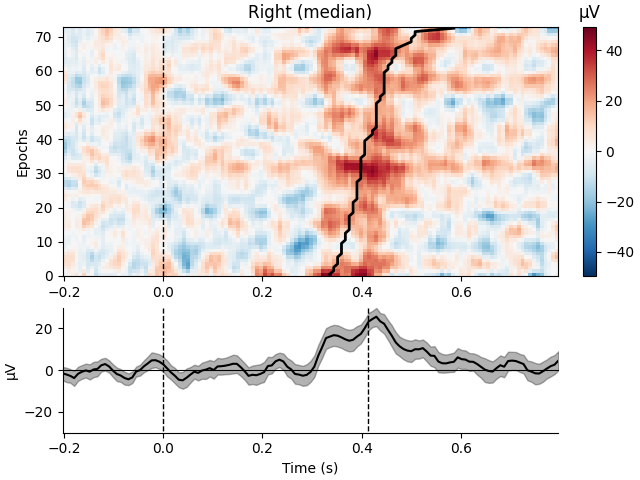Out:

```Loading data for 73 events and 129 original time points ...
73 matching events found
No baseline correction applied
0 projection items activated
73 matching events found
No baseline correction applied
0 projection items activated
73 matching events found
No baseline correction applied
0 projection items activated